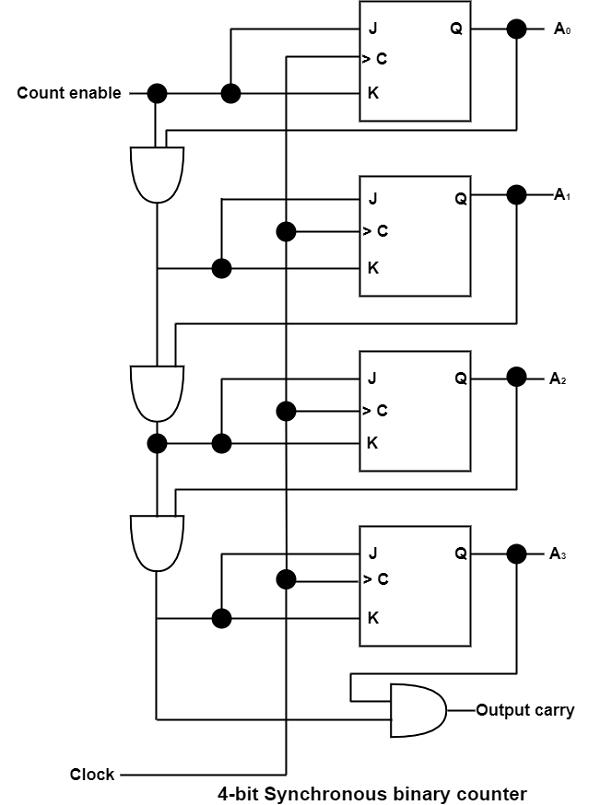# What are Binary Counters?

Computer ArchitectureComputer ScienceNetwork

A register that goes through a fixed series of states upon the software of input pulses is known as a counter. The input pulses can be clock pulses or can emerge from an outside source. They can appear at orderly intervals of time or at random.

Counters are initiate in almost all apparatus including digital logic. It can be used for counting the multiple occurrences of an event and are beneficial for producing timing signals to regulate the sequence of operations in digital computers. There are multiple sequences that a counter can follow and the consecutive binary sequence is clean and honest.

A counter that pursues the binary number sequence is known as a binary counter. An n-bit binary counter is a register of n flip-flops and related gates that follows a sequence of states as per the binary count of n bits, from 0 to 2" – 1.

A sequence of binary numbers including 0000, 0001, 0010, 0011, etc. The lower-order bit is achieved after each count and each other bit is achieved from one count to the next if and only if all its lower-order bits are similar to 1. For example, the binary count from 0111 (7) to 1000 (8) is appeared by (a) complementing the low-order bit, (b) complementing the second-order bit because the first bit of 0111 is 1, (c) complementing the third-order bit because the first two bits of 01 11 are 1's, and (d) complementing the fourth-order bit because the first three bits of 0111 are all 1's.

Synchronous binary counters have a normal pattern, as can be view from the 4-bit binary counter demonstrated in the diagram. The C inputs of all flip-flops obtain the common clock. If the count allow is 0, all J and K inputs are supported at 0 and the output of the counter does not modify.The first stage A0 is complemented when the counter is allowed and the clock goes through a positive transition. Each of the other three flip-flops is complemented when all preceding smallest significant flip-flops are similar to 1 and the count is allowed. The chain of AND gates creates the needed logic for the J and K inputs. The output carry can be used to develop the counter to further procedures, with each procedure having an extra flip-flop and an AND gate.